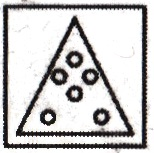• Save

# Paper Cutting and Folding Questions for Competitive Exams

Vikram Singh6 months ago 3.2K ViewsThe paper cutting and folding topic is related to the reasoning section. The questions asked in this topic, the given figure (paper) is folded, and then it is cut or folded according to the question. Then the shape obtained by opening it is one of the 4 to 5 options given in the answer figures. Candidates have to answer this option.

Here, I am getting you important questions related to paper cutting and folding under the Reasoning section in various competitive exams, which will help you to solve stressful questions in the exam and build a strong foundation. If you also want to bring the whole number in this topic, then try to solve the questions based on cutting and folding paper given here.

## Important Paper Cutting and Folding Questions

Q :

Direction: In the question figures below, a piece of paper is folded and cut as shown below. Find out from the answer figures how it will appear when opened.
Question figure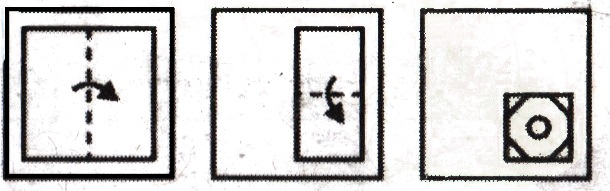(A)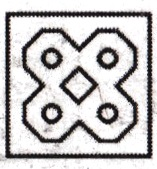(B)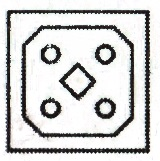(C)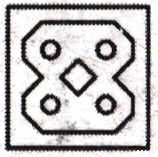(D)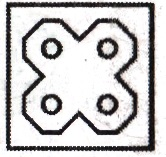Q :

Question figure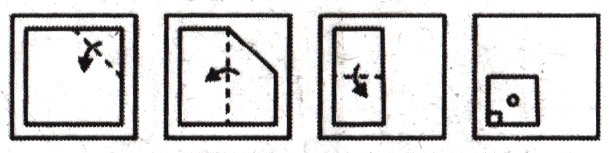(A)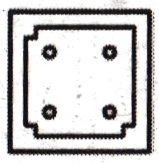(B)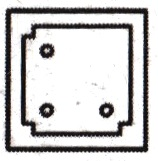(C)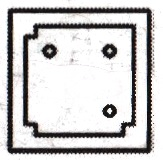(D)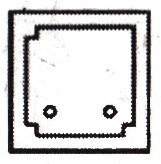Q :

Question Figure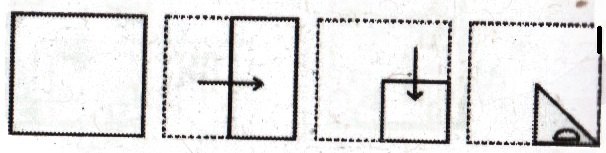(A)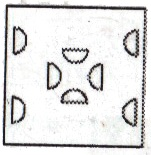(B)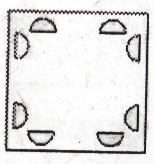(C)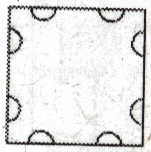(D)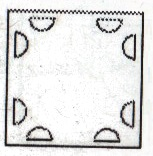Q :

Question Figure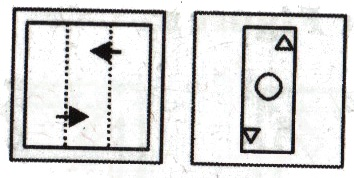(A)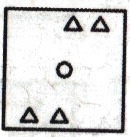(B)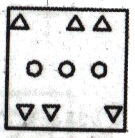(C)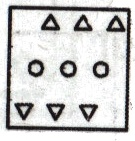(D)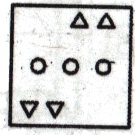Q :

Question figure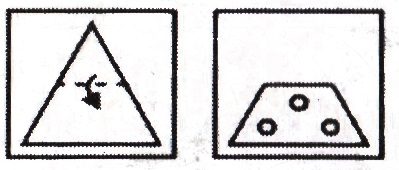(A)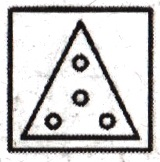(B)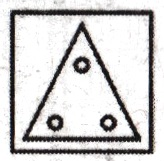(C)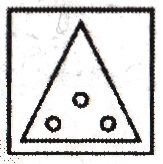(D)